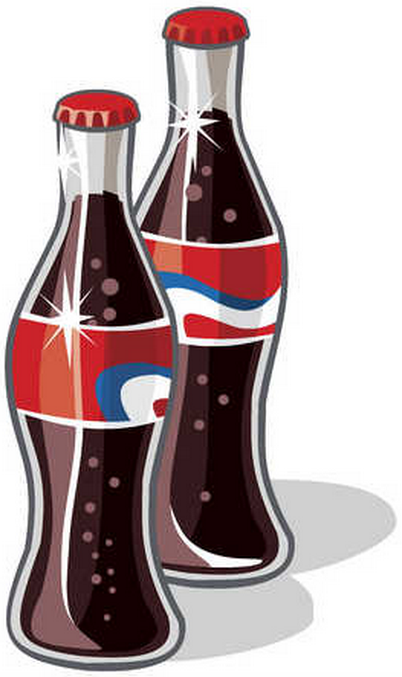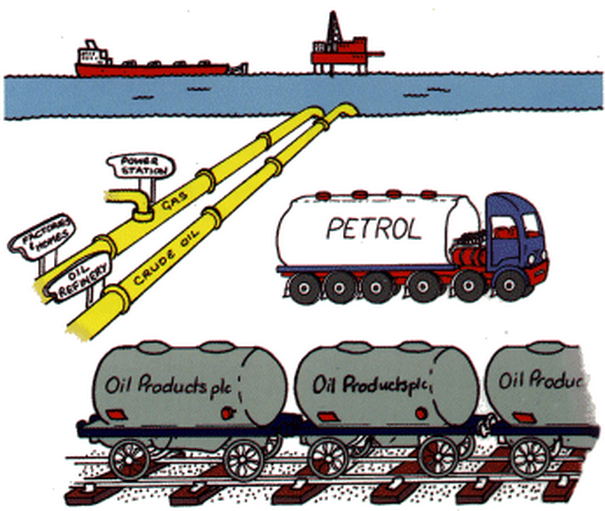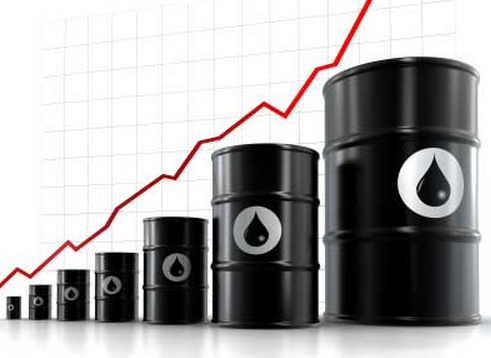# 5 ways we use liquid density informationSome background:

Simply put density is a physical property of matter. Density is expressed as a ratio of
mass to a given volume mathematically as Density = Mass / Volume. For our industrial
purposes and earth’s known gravitational pull we will define Mass as weight.  For liquids, temperature is an important factor that can affect a liquids density.  Therefore we generally will always describe density at a given temperature for comparison purposes. In general, as liquid temperature increases, density decreases.
Knowing the density of a liquid serves numerous purposes and can provide valuable information for making decisions. Understanding what density is, what affects density, and how density affects other physical properties is important in using and translating this information into an everyday useful tool.  We can use either a hand-held density meter or benchtop density meter to evaluate a liquids density or specific gravity.

##We live in a physical world and so it would make sense that we would want to be able
to define these physical properties around us. Having a way to define these physical
properties is helpful to those who need to use the information.
• >How much space does it take up?
• >How heavy is it?
• >How much of this can we put into that?
• >What is it?
• >Many everyday products are packaged by volume but sold by weight.
• >Predictive correlations can be made if you know the density.
• >If we change some of the ingredients that make up the fluid property will it change the weight?

#### The neat thing about understanding liquid density is that it can answer not only the three basic questions;

•>What is the mass of the liquid?,
• >what is the volume of the liquid?
• >What is the density of the liquid?

## 2. You can use a liquids density to predict

Fluid properties are probably even more telling where predictive
correlations can be made with density measurement information. Liquid
density changes as ingredients change. A soft-drink containing more sugar for example will have a different (higher) density than a diet soft-drink. Yes,
sugar not only increases a liquid’s density but can also be inversely measured
by knowing the liquids density in a given liquid solution. Understanding these
correlations provides useful information in the production of many beverages.

## 3. Density helps us better understand how to transport fluids

Moving fluids from point A to point B. Knowing the volume and the density
helps determine the best way of transporting those fluids. The answer might
be a bigger tanker truck, or for a pipeline it may be a larger transfer pump.## 4. Density helps set petroleum pricesPetroleum is used mostly by volume for the production of fuel, gasoline and
other energy sources. Density is used in petroleum production to give an
estimation of the gasoline or kerosene present thereby helping to determine
the price of the gasoline, for example.

In the oil industry quantities of crude oil are measured in metric tons.
Understanding the density allows one to calculate using API gravity the
number of barrels per metric ton –a common term of measure in the industry.## 5. Density as it relates to gravityTo further explore and expand the usefulness of density measurement we focus our attention on two types of gravity.

### Specific gravity

Specific gravity is another way of saying density relative to a given reference material.
In this case we usually mean relative density with respect to water. Water has a
density of 1,000 kg/m cubed at 4 degrees C. We say that water therefore has a specific
gravity of 1 (1,000/1,000). Specific gravity is the heaviness of a substance compared
to that of water. Have you ever noticed how common everyday automotive oil floats
on top of water? Automotive oil has an average density of 905 kg/m cubed at 15degrees C. We can calculate the specific gravity of the automotive oil by dividing 905
by 1,000 = 0.905. You can see that the number 0.905 is less than 1.0 and therefore is
lighter than water and is why this oil floats.
Looking at specific gravity another way, for those working in the production of wine, the density of
wine increases as you add sugar but falls as the yeast eats the sugar- finished wine should
have a specific gravity somewhere between .99 and 1.01.  Who knew?

### API gravity

The American Petroleum Institute (API) came up with their own gravity term referred
to as API gravity. API gravity is simply a measure of how heavy or light a petroleum
liquid is compared to water. By definition, if the petroleum’s API gravity is greater
than 10 then it is lighter than water and will float on water. If the API gravity is less
than 10 then it is heavier than water and will sink. The API gravity scale does not
have a unit of measure per say but is referred to in “degrees” with a scale from 10 to
70. This API gravity scale allows relative densities of petroleum liquids to be
compared. Why is this important? Why do we want to be able to compare petroleum
liquids? Well, the answer has to do with classifying the petroleum by quality. In the
oil industry crude oil is classified as either light, medium or heavy, according to it’s
measured API gravity! The measured API gravity will determine the class of oil and
therefore determine the value of the product.Light crude oil has an API gravity higher than 31.1 degrees API
Medium oil has an API gravity between 22.3 degrees API and 31.1 degrees API
Heavy oil has an API gravity below 22.3 degree API
Extra Heavy oil has an API gravity less than 10 degree API

CONCLUSION:

It is not always easy to understand how density may apply to a given circumstance but
you can hopefully see from this brief introduction that it plays a very important role in
many or our industrial and production processes.